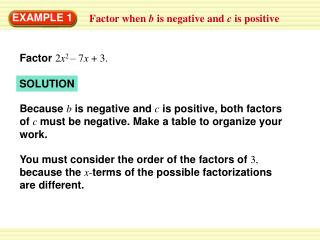Download PresentationEXAMPLE 1

# EXAMPLE 1

Télécharger la présentation## EXAMPLE 1

- - - - - - - - - - - - - - - - - - - - - - - - - - - E N D - - - - - - - - - - - - - - - - - - - - - - - - - - -
##### Presentation Transcript

1. EXAMPLE 1 Factor when b is negative and c is positive Factor 2x2 – 7x + 3. SOLUTION Because bis negative and cis positive, both factors of c must be negative. Make a table to organize your work. You must consider the order of the factors of 3, because the x-terms of the possible factorizations are different.

2. Factors of 2 Factors of 3 Possible factorization Middle term when multiplied 1,2 –1, –3 (x – 1)(2x – 3) –3x – 2x = –5x 1,2 3, 1 (x – 3)(2x– 1) –x – 6x = –7x Correct 2x2– 7x + 3 = (x – 3)(2x – 1) ANSWER EXAMPLE 1 Factor when b is negative and c is positive

3. EXAMPLE 2 Factor when b is positive and c is negative Factor 3n2 + 14n – 5. SOLUTION Because b is positive and cis negative, the factors ofc have different signs.

4. Factors of 3 Factors of –5 Possible factorization Middle term when multiplied 1, 3 1, –5 (n + 1)(3n – 5) –5n + 3n = – 2n 1, 3 –1, 5 (n – 1)(3n + 5) 5n – 3n = 2n 1, 3 5, –1 (n + 5)(3n –1) –n + 15n = 14n Correct 1, 3 –5, 1 (n – 5)(3n + 1) n – 15n = –14n 3n2 + 14n – 5 = (n + 5)(3n – 1) ANSWER EXAMPLE 2 Factor when b is negative and c is positive

5. ANSWER (t + 2)(3t + 2) ANSWER (s – 1)(4s – 5) ANSWER (h + 7)(2h – 1) for Examples 1 and 2 GUIDED PRACTICE Factor the trinomial. 1. 3t2 + 8t + 4 2. 4s2 – 9s + 5 3.2h2 + 13h – 7